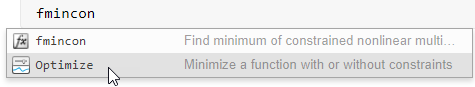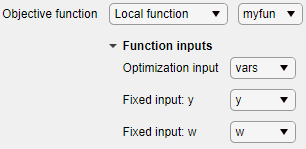# Optimize

Optimize or solve equations in the Live Editor

Since R2020b

## Description

The Optimize task lets you choose between two ways to interactively optimize problems or to solve nonlinear systems of equations:

• Problem-based (recommended) — Create symbolic optimization variables and expressions to represent the objective function and constraints or equations.

• Solver-based — Represent the objective function and constraints or equations using standard MATLAB® code.

Using the problem-based version of this task, you can:

• Specify optimization variable arrays, including their bounds and initial values.

• Specify the problem type: minimization, maximization, feasibility, or equation-solving.

• Specify the objective and constraint functions, either by writing expressions or browsing for functions.

• Optionally, choose a solver, and specify nondefault options.

• Run the optimization.

Using the solver-based version of this task, you can:

• Choose a solver based on the characteristics of your problem. If you have Global Optimization Toolbox, you can choose to use its solvers as well.

• Specify the objective and constraint functions, either by writing functions or browsing for functions.

• Specify solver options.

• Run the optimization.

To get started using Optimize, see Get Started with Solver-Based Optimize Live Editor Task and Get Started with Problem-Based Optimize Live Editor Task. For suggestions on how to use Optimize, see Use Solver-Based Optimize Live Editor Task Effectively or Use Problem-Based Optimize Live Editor Task Effectively. Currently, you cannot use the `fseminf`, `GlobalSearch`, or `MultiStart` solvers with Optimize.

To add the Optimize task to a live script in the MATLAB Editor, on the Live Editor Insert tab, select Task > Optimize.Alternatively, in a code block in the script, type a relevant keyword, such as `optim` or `fmincon`. Select Optimize from the suggested command completions.## Parameters

expand all

The problem-based variable names are dynamic; you can choose any names you like subject to the usual naming restrictions (see Variable Names).

Problem-Based

Problem type, specified by clicking the appropriate labeled button.

Objective function, specified as an optimization expression, a local function, or a function file. Applies when the `Goal` is `Minimize` or `Maximize`.

Constraints on solution, specified as an optimization expression, local function, or function file. Add constraints to the problem by clicking the + button.

Problem equations, specified as an optimization expression, local function, or function file. Applies when the `Goal` is ```Solve equations```. Add equations to the problem by clicking the + button.

State of task, specified as `Define problem` or ```Solve problem```. For more information, see Use Problem-Based Optimize Live Editor Task Effectively.

Solver-Based

Objective function type, specified by clicking the appropriate labeled button. The selected objective function determines which solvers are available and which solver is recommended for the problem (see `Solver`).

Constraint types, specified by clicking the appropriate labeled buttons. You can specify more than one constraint type. The selected constraints determine which solvers are available and which solver is recommended for the problem (see `Solver`).

Optimization solver that MATLAB uses to solve the problem, specified by selecting a solver from the list of available solvers. The available solvers and the recommended solver depend on your license and the selected `Objective` and `Constraints`.

## Limitations

• Currently, Optimize has the following restrictions for multiobjective optimization.

• You must specify your objective functions using a single function with multiple outputs. In other words, your objective function must output a vector of values, one entry for each objective.

• All objective functions must use the same sense, minimization for the solver-based task, and either minimization or maximization for the problem-based task.

## Tips

• For functions with extra inputs, the solver-based and problem-based Optimize tasks have somewhat different requirements.

• Solver-Based: Choose the optimization variable, and specify which workspace variables contain the fixed data inputs. For example, see Place Optimization Variables in One Vector and Data in Other Variables, which contains three function inputs:Optimize generates code only after you specify all function inputs.

• Problem-Based: Specify an optimization variable or workspace variable name for each function input. If an input argument name in the function signature matches an existing optimization variable or workspace variable name, Optimize automatically selects that name.Optimize generates code only after you specify all function inputs.

• Optimize cannot parse a function containing the `varargin` input or a function that contains an error.

• If you select a function from a file, Optimize adds the file location to your MATLAB path.

• If Optimize has a parsing error or if multiple local functions have the same name, the list of available local functions is empty.

## Version History

Introduced in R2020b